In two recent RPubs adventures, this one here and that one there, we were working with ggplot examples inspired by Hadley Wickham’s book, R for Data Science.

Going through our examples, we found all relied on three common arguments: data, geom_, and aes. The idea driving ggplot is that any graph you can imagine can be depicted using a set of layers, these three being the most basic. Altogether, seven layers can be used in ggplot, but the three mentioned above are the minimum needed for ggplot to work.

The seven layers constitute what Wickham calls “the layered grammar of graphics.”

1. data, the data frame you want to plot
2. a geom_function maps out the x and y values and how to represent them
3. the aes argument specifies the visual properties you wish to use
4. the stat_function allows you to use statistical depictions of data
5. position determine where elements are situated relative to each other
6. a coordinate_function manipulates the coordinate system used to graph
7. the two facet_functions display multiple versions of the graph

There can be many types of each element. For example, there are nearly 30 geom_functions. The ggplot provides a lot of flexibility for designing visuals for data. And the whole thing works because of layering.

You can get an idea of layering below. You can find the code here on GitHub.

``````library(ggplot2)
library(maps)``````

Using the three basic arguments we can create a rudimentary graph of our data. In this case, it’s nothing fancy, just a simple bar graph, as specified by the geom_bar argument.

``ggplot(data = diamonds) + geom_bar(mapping = aes(x = cut))``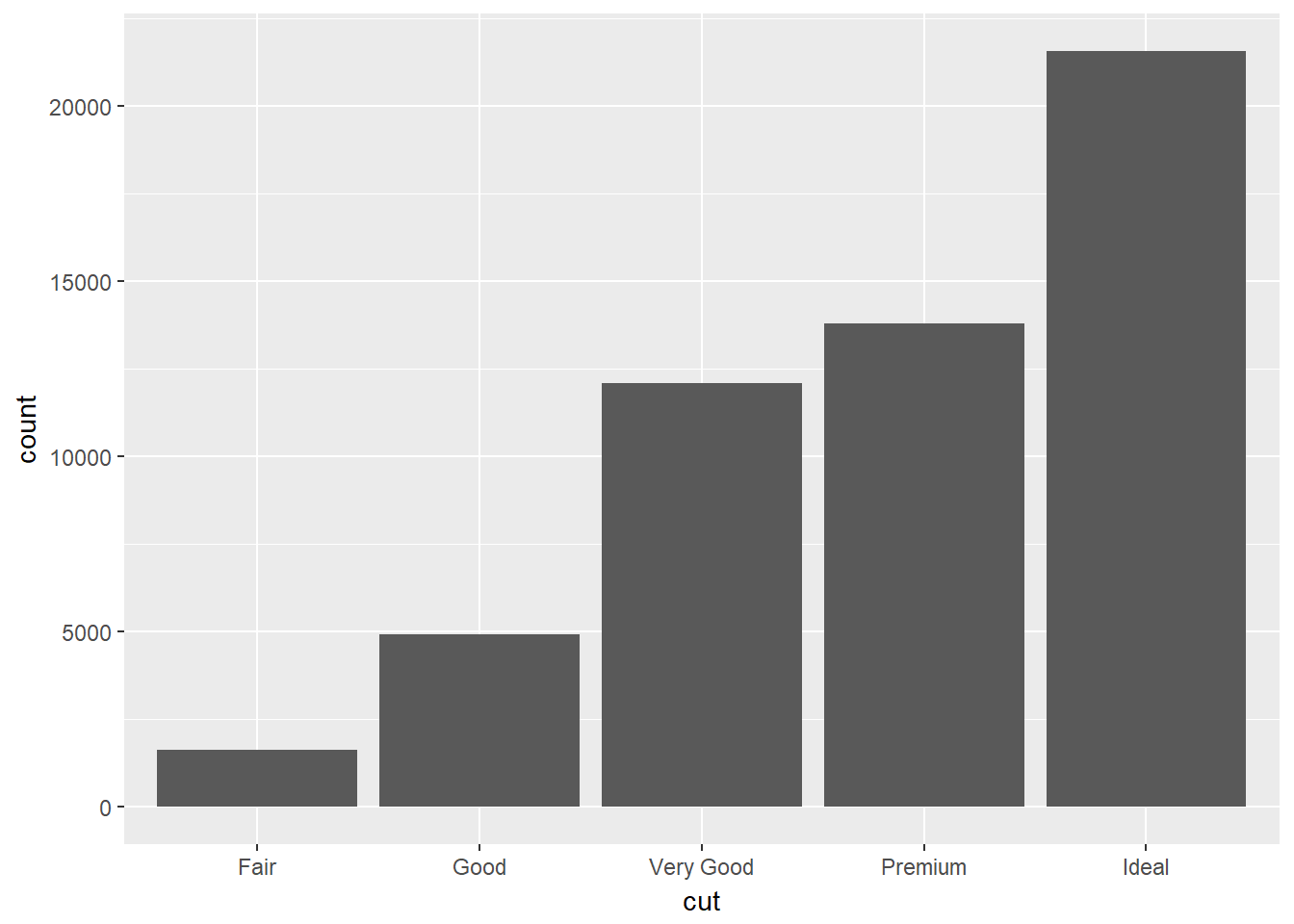We can create the same graph by using the stat_count argument instead of geom_bar. We can’t tell a lot about the data just by looking at this graph. We need to add more information, more layers, in order to communicate better. To start, we’ve added a title to make it a bit more useful.

``ggplot(data = diamonds) + stat_count(mapping = aes(x = cut)) + ggtitle("Diamond Cuts by Quality by Count")``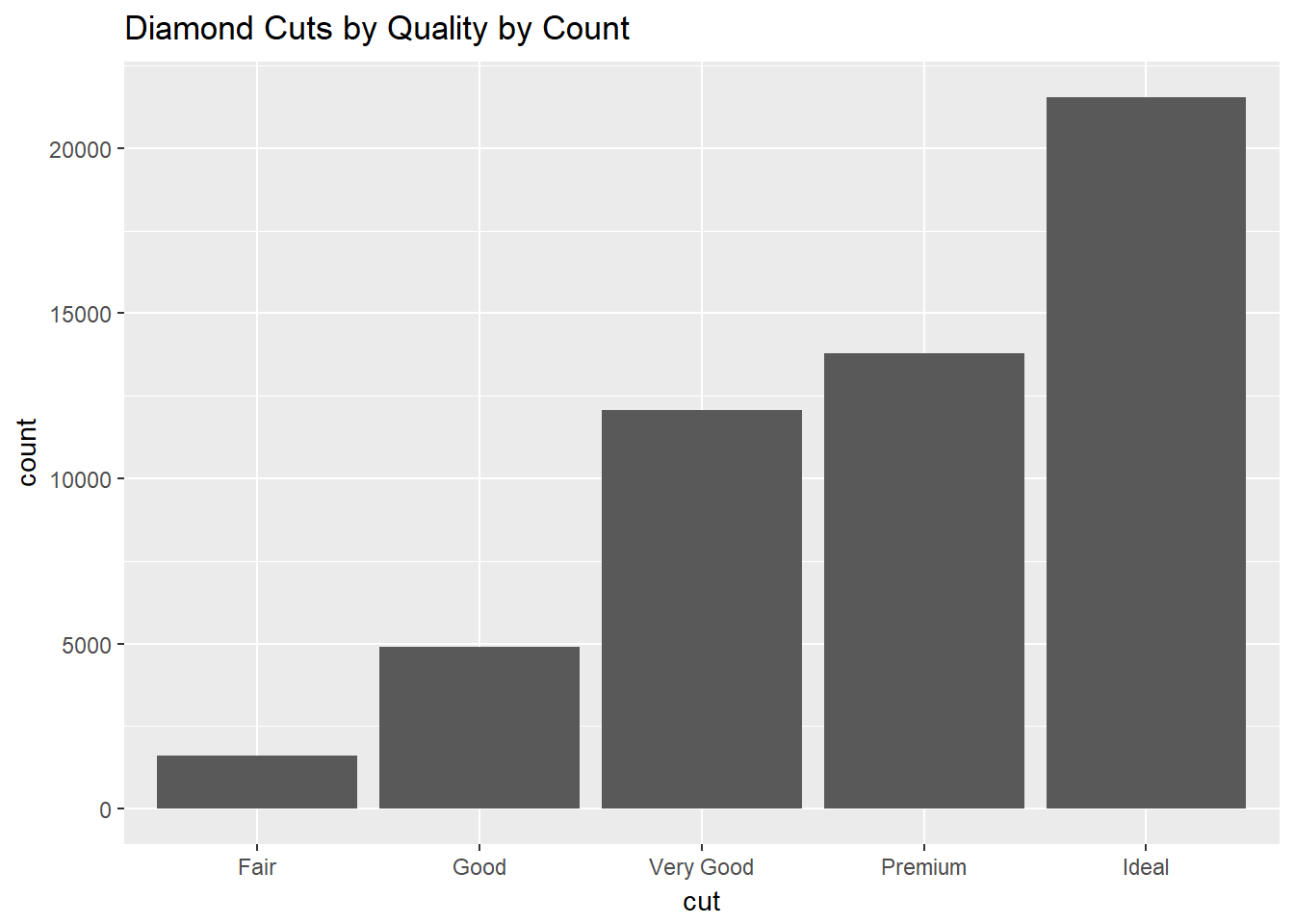The previous plots were based on counts of diamonds in each bin, but we can also express the y-value in terms of percentages.

``````ggplot(data = diamonds) + geom_bar(mapping = aes(x = cut, y = ..prop.., group = 1)) +
ggtitle("Diamond Cuts by Quality by Percent")``````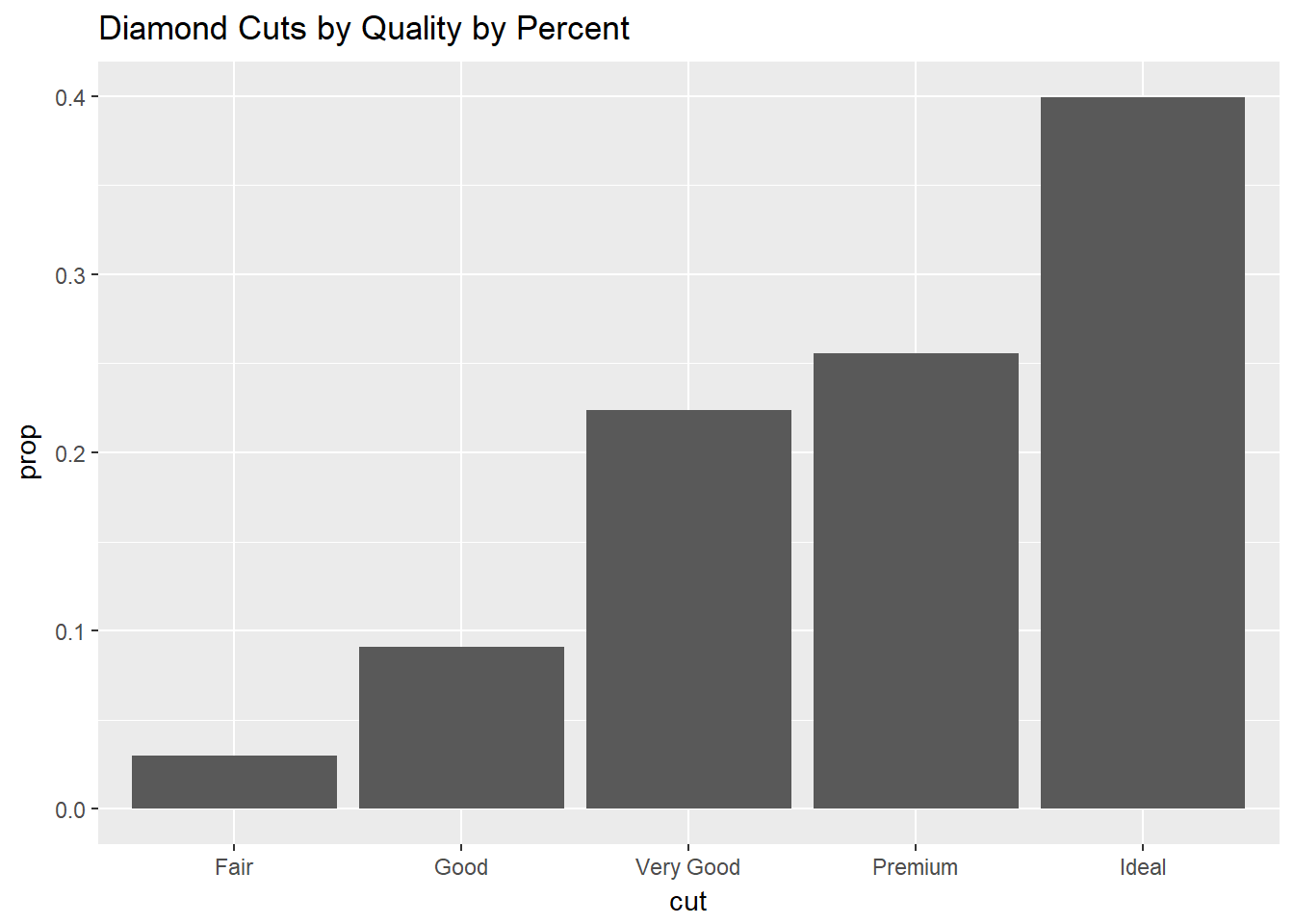We can map values to different arguments to change the appearance of the graph. Here, we use stat_summary to map the data and then limit the expression to the minimum, maximum, and median values for each class. We are no longer working with bar graph geometry.

``````ggplot(data = diamonds) + stat_summary(mapping = aes(x = cut, y = depth), fun.ymin = min, fun.ymax = max, fun.y = median) +
ggtitle("Diamond Cuts by Quality by Depth")``````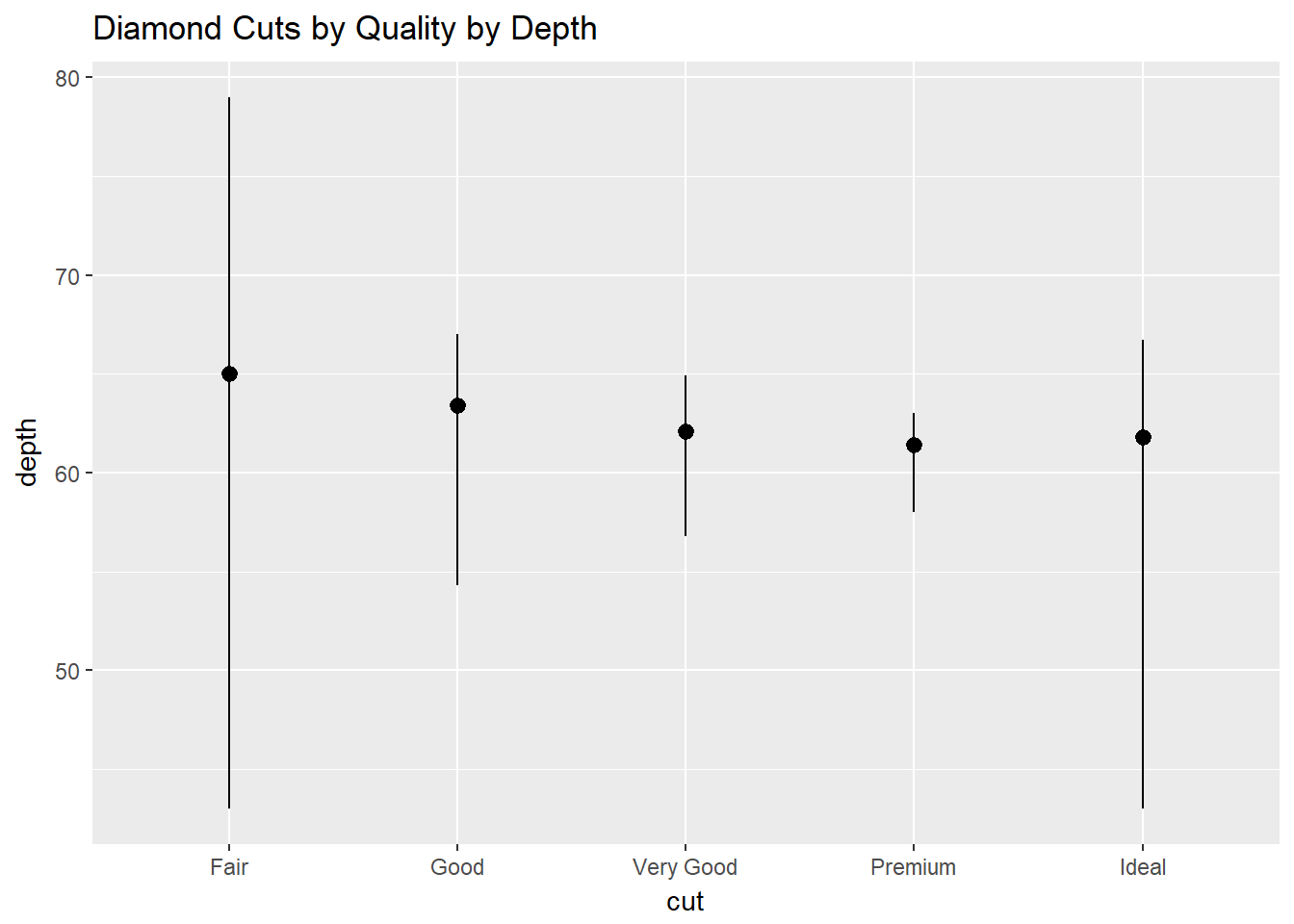Adding color to the chart isn’t difficult, but we have to distinguish how it is to be used. Here, we use color to outline the gray bars.

``````ggplot(data = diamonds) + geom_bar(mapping = aes(x = cut, color = cut)) +
ggtitle("Diamond Cuts")``````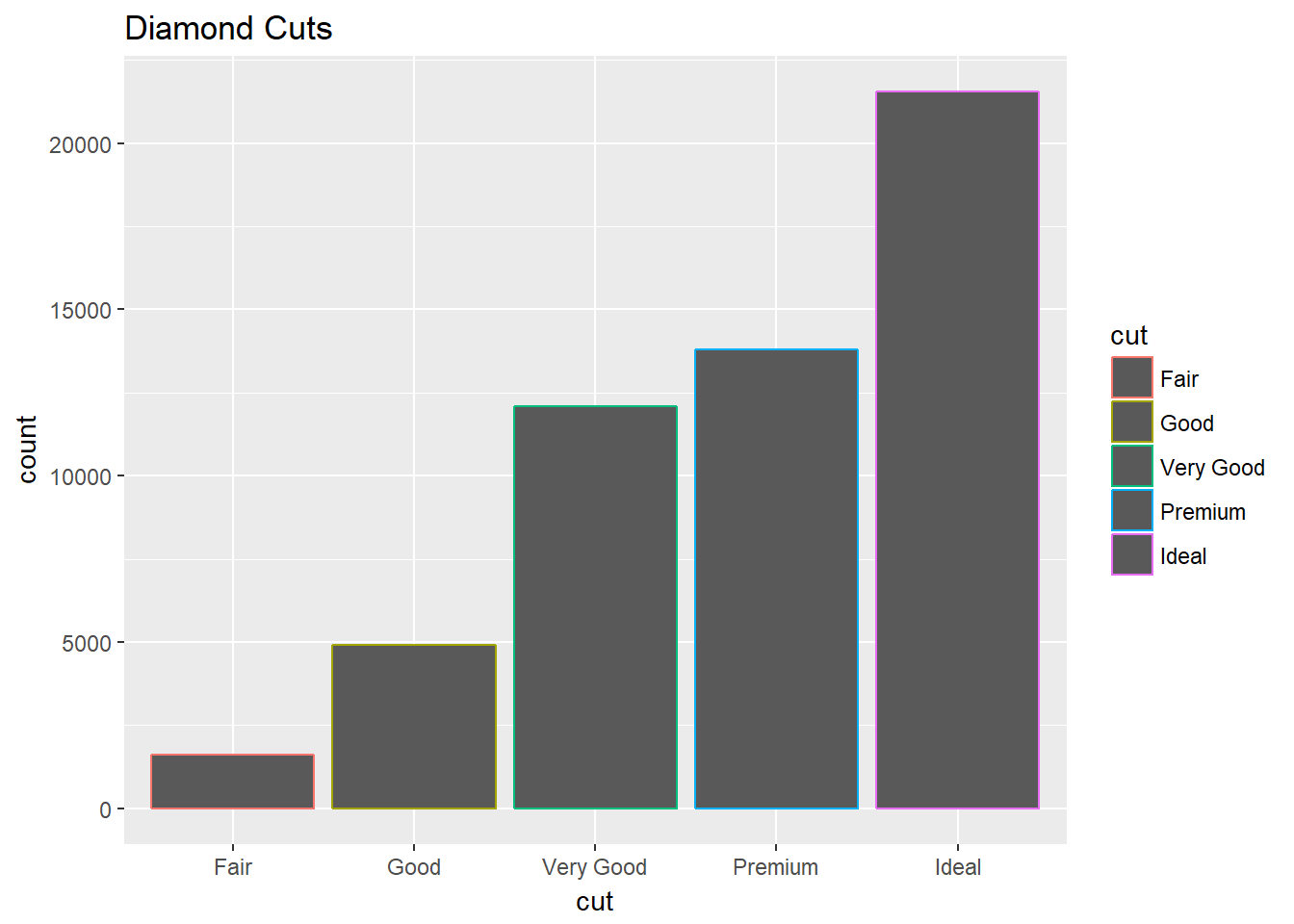Or we could use color to fill the bars.

``````ggplot(data = diamonds) + geom_bar(mapping = aes(x = cut, fill = cut)) +
ggtitle("Diamond Cuts")``````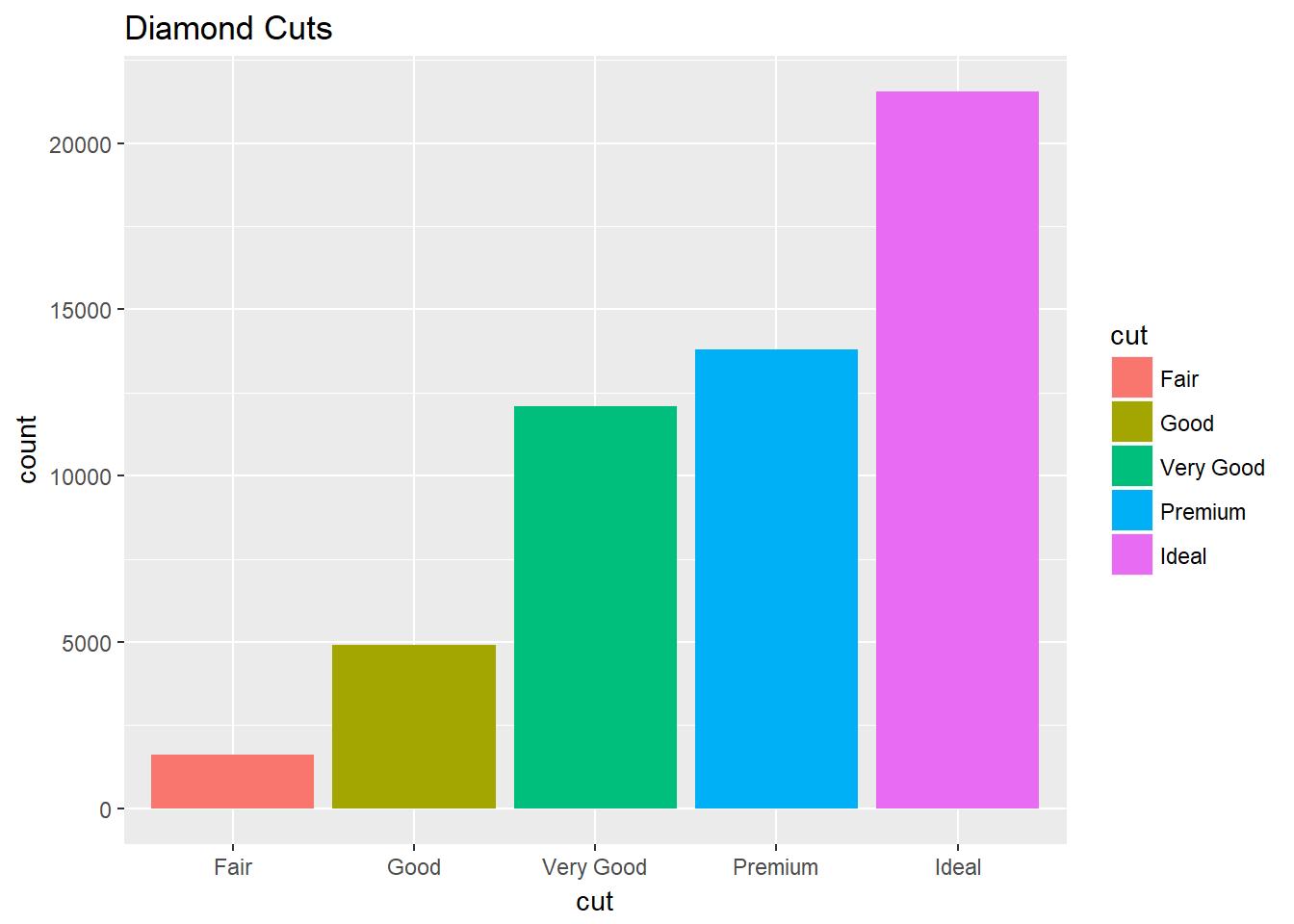We can stack the bars in order to add a third variable to our graph. Here we look at each cut class by its clarity.

``````ggplot(data = diamonds) + geom_bar(mapping = aes(x = cut, fill = clarity)) +
ggtitle("Diamonds by Cut and Clarity")``````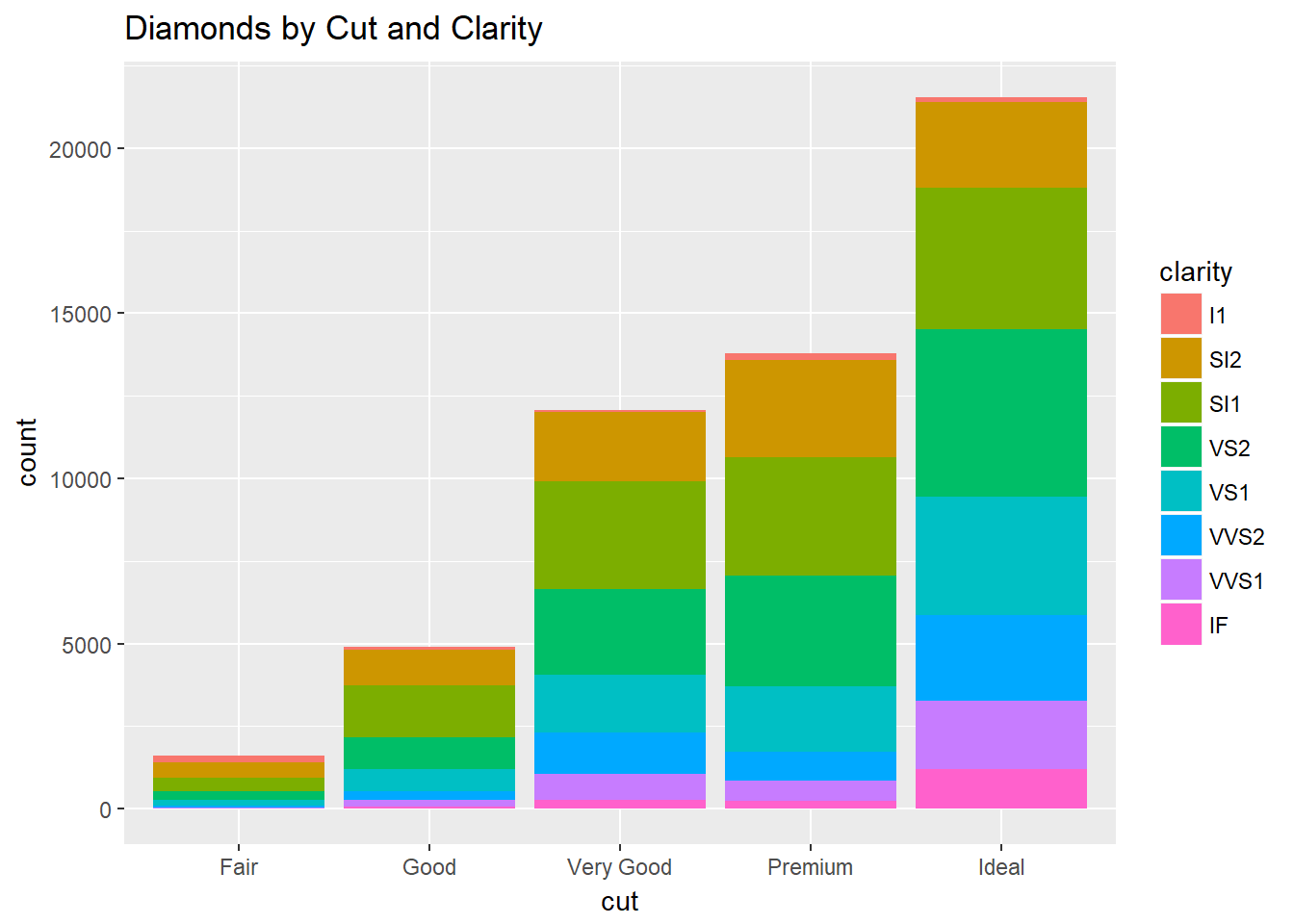A twist on the stacked theme is to work with proportions rather than counts. You see how position can be used.

``````ggplot(data = diamonds, mapping = aes( x = cut, fill = clarity)) +
geom_bar(position = "fill") + ggtitle("Diamond Cuts by Clarity and Percent")``````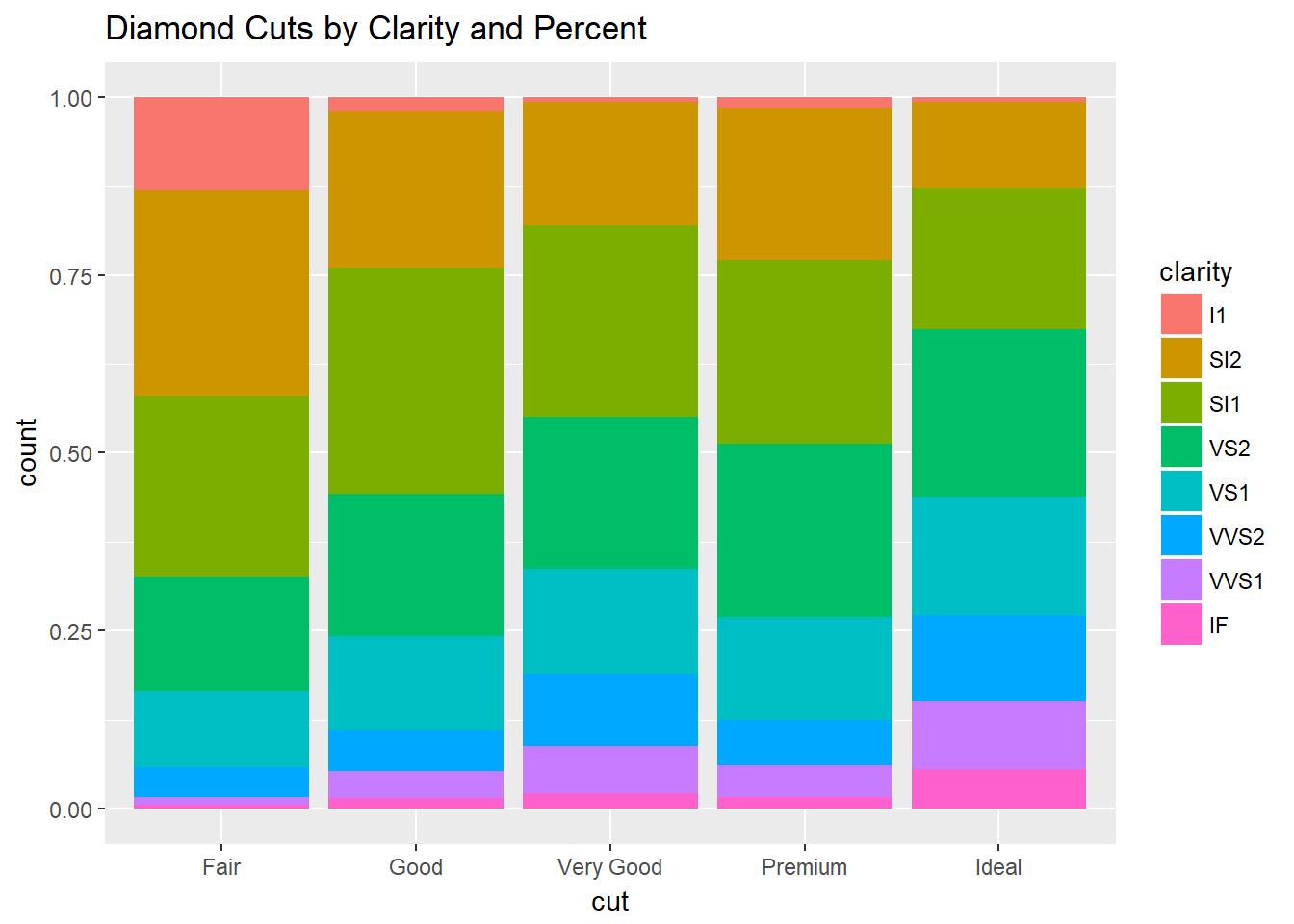Rather than stacking, another way to depict the classes is to arrange bars side-by-side. Again, position is employed.

``````ggplot(data = diamonds, mapping = aes( x = cut, fill = clarity)) +
geom_bar(position = "dodge") + ggtitle("Diamond Cuts by Clarity")``````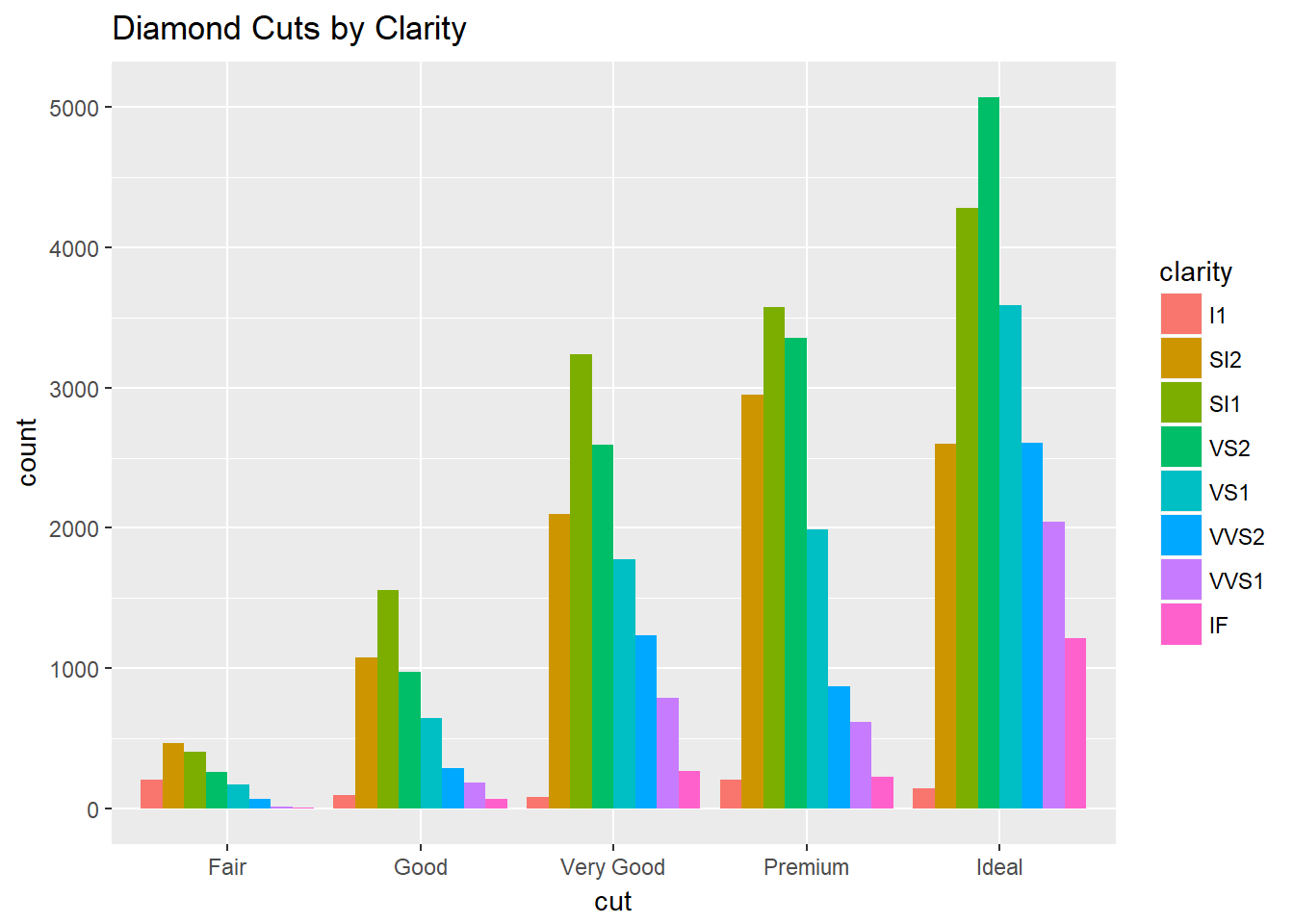We are going to illustrate the use of the coordinate_function by going back to an example from our mpg data set. First, we use a boxplot geometry in the ordinary x-y orientation, with the x-axis running along the bottom. As we’d expect, the boxplots are arranged vertically.

``````ggplot(data = mpg, mapping = aes(x = class, y = hwy)) + geom_boxplot() +
ggtitle("Highway Mileage by Class") +
labs(x = "vehicle class", y = "highway mileage/gallon")``````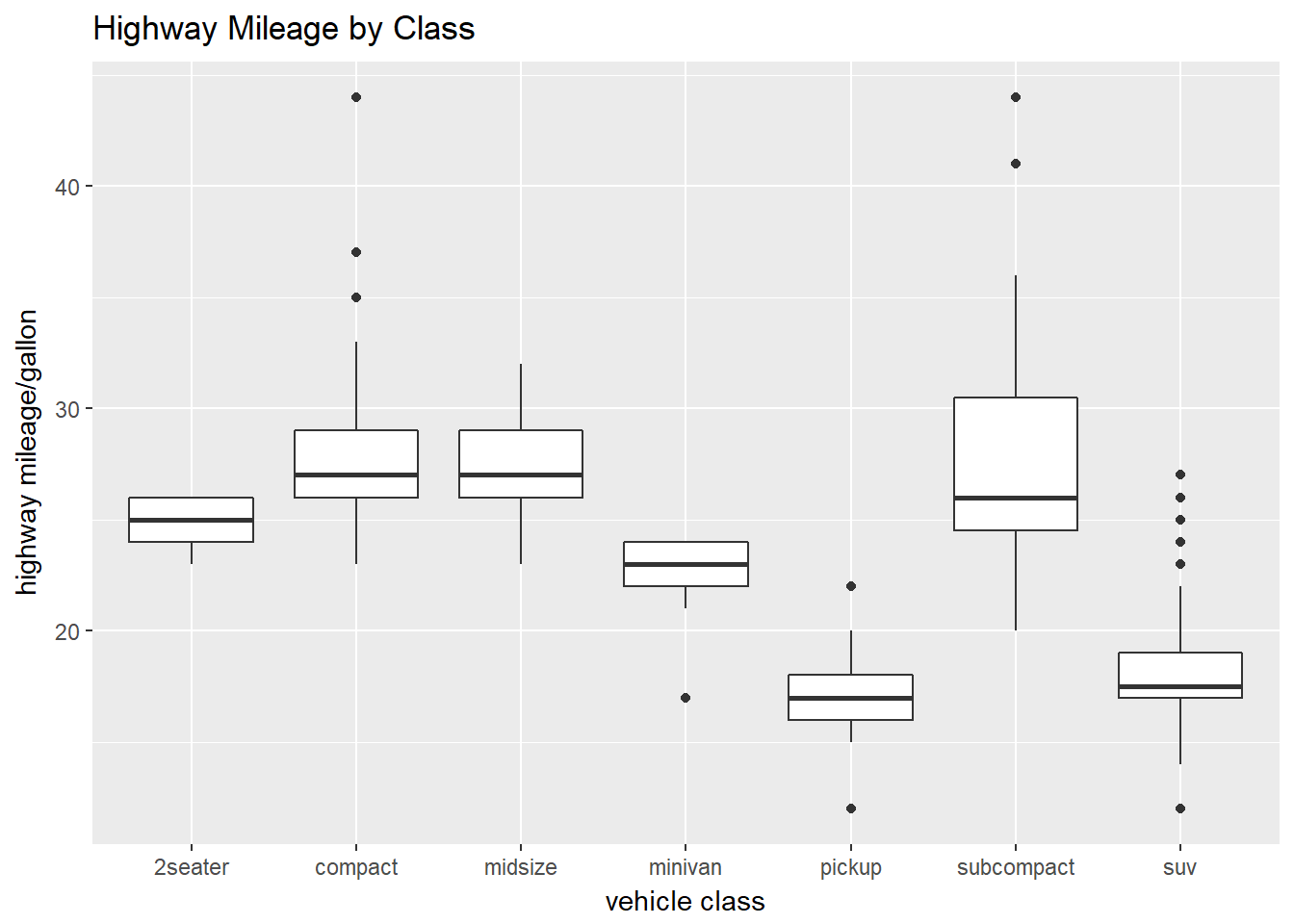But if we would like to show the boxplots horizontally, we flip the coordinates around and put the y-axis along the bottom. To do this, use the coord_flip argument.

``````ggplot(data = mpg, mapping = aes(x = class, y = hwy)) + geom_boxplot() + coord_flip() +
ggtitle("Highway Mileage by Class") +
labs(x = "vehicle class", y = "highway mileage/gallon")``````